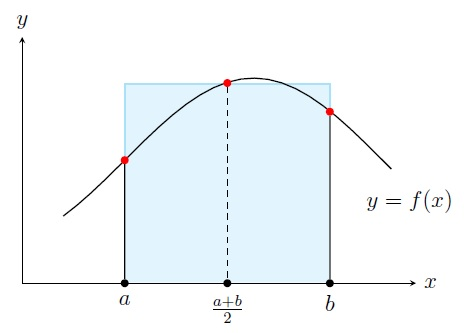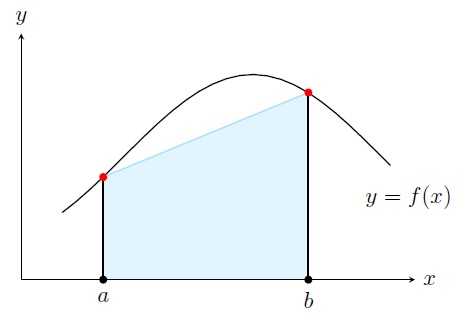Numerical Analysis Home

## Elements of Numerical Integration

Sometimes it is hard to calculate a definite integral analytically. For example, $$\displaystyle\int_{0}^1 e^{x^2} \,dx$$. To approximate such an integral we break $$[a,b]$$ into $$n$$ subintervals $$[x_0,x_1],[x_1,x_2],\ldots,[x_{n-1},x_n]$$ where $$x_i=a+ih$$ and $$h=(b-a)/n$$. Then we approximate the integral by a finite sum given by a quadrature rule (or, quadrature formula): $\int_{a}^{b} f(x) \,dx \approx \sum_{i=0}^n c_if(x_i).$ A quadrature rule you have seen before is the Midpoint Rule: $\int_{a}^{b} f(x) \,dx \approx (b-a) f \left(\frac{a+b}{2} \right).$ It approximates the area given by $$\int_{a}^{b} f(x) \,dx$$ by the area of the rectangle with length $$(b-a)$$ and width $$f \left(\frac{a+b}{2} \right)$$.Let's discuss other quadrature rules. Recall that we can approximate $$f(x)$$ by the Lagrange polynomial $$P_n(x)$$ of degree $$n$$ using $$n+1$$ points $$a=x_0,x_1\ldots,x_n=b$$: $f(x)\approx \sum_{i=0}^n f(x_i)L_i(x),$ where $$L_i(x)=\displaystyle\prod_{\substack{j=0\\j\neq i}}^n\frac{(x-x_j)}{(x_i-x_j)}$$. Integrating both sides, we get \begin{align*} & \int_{a}^b f(x)\,dx \approx \int_{a}^b\sum_{i=0}^n f(x_i)L_i(x) \,dx= \sum_{i=0}^n f(x_i) \left[\int_{a}^b L_i(x) \,dx \right] \end{align*} $\implies \int_{a}^b f(x)\,dx \approx \sum_{i=0}^n c_i f(x_i),$ where $$c_i=\int_{a}^b L_i(x) \,dx$$. We will discuss the quadrature rules given by $$n=1$$ and $$2$$.

For $$n=1$$, we have $$n+1=2$$ points $$a=x_0,x_1=b$$ and then $\begin{array}{lrl} & f(x) &\approx P_1(x)=f(x_0) \displaystyle\frac{(x-x_1)}{(x_0-x_1)}+f(x_1)\frac{(x-x_0)}{(x_1-x_0)}=-f(a)\frac{(x-b)}{(b-a)}+f(b)\frac{(x-a)}{(b-a)}\\ \implies & \displaystyle\int_{a}^b f(x)\, dx &\approx -\displaystyle\frac{f(a)}{b-a} \int_{a}^b (x-b)\, dx+ \frac{f(b)}{b-a} \int_{a}^b (x-a)\, dx\\ & &= -\displaystyle\frac{f(a)}{b-a} \left.\frac{(x-b)^2}{2} \right]_{a}^b + \frac{f(b)}{b-a} \left.\frac{(x-a)^2}{2} \right]_{a}^b \\ & &= (b-a)\left[\displaystyle\frac{f(a)+f(b)}{2}\right] \end{array}$ So we get the Trapezoidal Rule: $\boxed{\int_{a}^{b} f(x) \,dx \approx (b-a)\left[\displaystyle\frac{f(a)+f(b)}{2}\right]}$ It approximates the area given by $$\int_{a}^{b} f(x) \,dx$$ by the area of the trapezoid with height $$(b-a)$$ and bases $$f(a)$$ and $$f(b)$$.The error in the trapezoidal rule is the integral of the error term for the Lagrange polynomial: $E_T=\int_a^b \frac{f''(\xi(x))}{2!}(x-a)(x-b)\, dx.$ By the Weighted MVT (where $$f''(\xi(x))$$ is continuous and $$(x-a)(x-b)$$ does not change sign in $$[a,b]$$), we get a constant $$c\in (a,b)$$ such that $E_T= \frac{f''(c)}{2}\int_a^b (x-a)(x-b)\, dx=-f''(c)\frac{(b-a)^3}{12}.$ Similarly for $$n=2$$, we have $$n+1=3$$ points $$a=x_0,x_1=(a+b)/2,x_2=b$$ and then $f(x) \approx P_2(x)=f(x_0) \displaystyle\frac{(x-x_1)(x-x_2)}{(x_0-x_1)(x_0-x_2)}+f(x_1)\frac{(x-x_0)(x-x_2)}{(x_1-x_0)(x_1-x_2)}+f(x_2)\frac{(x-x_0)(x-x_1)}{(x_2-x_1)(x_2-x_1)}.$ Integrating we get the Simpson's Rule: $\boxed{\int_{a}^{b} f(x) \,dx \approx \frac{(b-a)}{6}\left[f(a)+4f\left(\frac{a+b}{2}\right)+f(b) \right]}$ where the error in the Simpson's Rule (obtained from the Taylor polynomial $$T_3(x)$$ of $$f$$ about $$x=(a+b)/2$$ with the error term) is $E_S= -\frac{(b-a)^5}{90\cdot 2^5}f^{(4)}(c).$ Note from $$E_T$$ that if $$f(x)$$ is a polynomial of degree at most 1, then $$f''=0$$ and consequently $$E_T=0$$. So the trapezoidal rule gives the exact integral. Similarly if $$f(x)$$ is a polynomial of degree at most 3, then $$E_S=0$$ and consequently the Simpson's rule gives the exact integral.

Example. Approximate $$\displaystyle\int_{0}^2 x^3\,dx$$ by the Midpoint Rule, Trapezoidal Rule, Simpson's Rule.

Solution. First of all let's find the exact integral: $\displaystyle\int_{0}^2 x^3\,dx= \left.\frac{x^4}{4}\right]_0^2=4.$ \begin{align*} \text{Midpoint}:\; \int_{0}^2 x^3\,dx &\approx (b-a) f \left(\frac{a+b}{2} \right)=(2-0) \left(\frac{0+2}{2} \right)^3= 2\\ \text{Trapezoidal}:\; \int_{0}^2 x^3\,dx &\approx (b-a)\left[\displaystyle\frac{f(a)+f(b)}{2}\right]=(2-0)\left[\displaystyle\frac{0+2^3}{2}\right]=8\\ \text{Simpson's}:\; \int_{0}^2 x^3\,dx &\approx \frac{(b-a)}{6}\left[f(a)+4f\left(\frac{a+b}{2}\right)+f(b) \right]= {\tiny\frac{(2-0)}{6} \left[0+4\cdot 1^3+2^3 \right]}=4 \end{align*} The Simpson's Rule gives the best approximation which turns out to be the exact integral.

Note that the error of the Midpoint Rule is always half of that of the Trapezoidal Rule. Because the Midpoint Rule is obtained by integrating the Taylor polynomial $$T_1(x)$$ of $$f$$ about $$x=(a+b)/2$$ and integrating its remainder term, we can show that $E_M= \frac{(b-a)^3}{24}f''(c).$

Last edited